Railroad-Highway Grade Crossing Handbook - Revised Second Edition August 2007 Appendix F: New Hampshire Hazard Index, NCHRP Report 50 Accident Prediction Formula, Peabody-Dimmick Accident Prediction Formula Table of Contents | Previous | Next

APPENDIX

F

New Hampshire Hazard Index,

NCHRP Report 50 Accident Prediction Formula, Peabody-Dimmick Accident Prediction Formula

The New Hampshire Index is as follows:

HI = (V) (T) (Pf)                                                         (1)

where:

HI = hazard index

V = annual average daily traffic

T = average daily train traffic

Pf = protection factor

= 0.1 for automatic gates

= 0.6 for flashing lights

= 1.0 for signs only

Several modifications of the New Hampshire Index are in use. Some states use various other values for Pf as follows:

•    0.13 or 0.10 for automatic gates.

•    0.33, 0.20, or 0.60 for flashing lights.

•    0.67 for wigwags .

•    0.50 for traffic signal preemption.

•    1.00 for crossbucks.

One state adds 1 to average daily train traffic (T). Several states use a hazard index that basically incorporates the New Hampshire Index but also includes other factors:

•    Train speed.

•    Highway speed.

•    Sight distance.

•    Crossing angle.

•    Crossing width.

•    Type of tracks.

•    Surface type.

•    Population.

•    Number of buses.

•    Number of school buses.

•    Number of tracks.

•    Surface condition.

•    Nearby intersection.

•    Functional class of highway.

•    Vertical alignment.

•    Horizontal alignment.

•    Number of hazardous material trucks.

•    Number of passengers.

•    Number of accidents.

Some of these hazard indices are shown in the following table:A5 = Number of accidents in five years SBP = Number of school bus passengers P = Factor for population Aa = Number of accidents per year P = Protection factor Af = Accident factor SD = Factor for sight distance AL = Factor for highway alignment T = Average number of trains per day AN = Factor for approach angle RF = Factor for rideability FC = Factor for functional class Tf = Number of fast trains G = Factor for approach grades T = Number of slow trains HI = Hazard Index T = Train factor HM = Factor for hazardous materials vehicles TN = Factor for number of night trains TR = Factor for number and type of tracks HS = Factor for highway speed TS = Factor for train speeds L = Factor for number of lanes TT = Factor for type of train movements LI = Factor for local interference TTR = Factor for type of tracks LP = Factor for local priority V = Annual average daily traffic S = Factor for surface type V = Factor for annual average daily traffic SB = Number of school buses VSD = Factor for vertical sight distance NTR = Factor for number of tracks W = Factor for crossing width

Source: Railroad-Highway Grade Crossing Handbook, Second Edition. Washington, DC: U.S. Department of Transportation, Federal Highway Administration, 1986.

National Cooperative Highway Research Program Report 50 Accident Prediction Formula

The hazard index presented in National Cooperative Highway Research Program (NCHRP) Report 50 can be expressed as a complex formula or reduced to a more simple equation of coefficients that are taken from a few tables and graphs. The simple formula for calculating the expected number of accidents per year is:

 Expected Accident Frequency = A x B x Current Trains per Day EXAMPLE ASSUME Vehicles Per Day (10 yr. ADT) A Factor 250-------------------.000347 500-------------------.000694 1000-------------------.001377 2000-------------------.002627 3000-------------------.003981 4000-------------------.005208 5000-------------------.006516 6000-------------------.007720 7000-------------------.009005 8000-------------------.010278 9000-------------------.011435 10000-------------------.012674 12000-------------------.015012 14000-------------------.017315 16000-------------------.019549 18000-------------------.021736 20000-------------------.023877 25000-------------------.029051 30000-------------------.034757 Urban area Crossbucks 5000 vehicles per day 5 trains per day EXPECTED ACCIDENT FREQUENCY EAF = .006516 x 3.06 x 5 EAF = 0.10 EAF = 1 accident every ten years Accident frequency is greater than 0.02. This would indicate need for higher type device Try flashing lights B = .23 EAF = .006516 x .23 x 5 EAF = 0.01 THEREFORE FLASHING LIGHTS ARE WARRANTED ‘B’ FACTOR COMPONENTS (‘B’ FACTOR BASIC VALUE ADJUSTMENTS) BASIC VALUES FOR EXISTING DEVICES A Crossbucks, highway volume less than 500 per day 3.89 B Crossbucks, urban 3.06 C Crossbucks, rural 3.08 D Stop signs, highways volume less than 500 per day 4.51 E Stop signs 1.15 F Wigwags 0.61 G Flashing lights, urban 0.23 H Flashing lights, rural 0.93 I Gates, urban 0.08 J Gates, rural 0.19

Source: Railroad-Highway Grade Crossing Handbook, Second Edition. Washington, DC: U.S. Department of Transportation, Federal Highway Administration, 1986.

NCHRP 50 also provides formulae for estimating the number of non-train-involved accidents per year as follows:

Automatic gates:All other traffic control devices: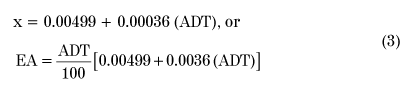100 where:

X = probability of coincidental vehicle and train arrival scaled by 10-3 ADT = average daily traffic EA = expected number of accidents per year

Modifications of the hazard index exist. State’s formula is: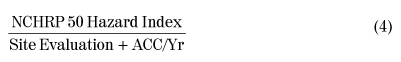The Site Evaluation factor is based on the following:

•    Most restrictive sight distance of all quadrants.

•    Crossing angle.

•    Distraction from traffic control devices.

•    People factor.

Each factor is rated from 1 (best) through 5 (worst), and the average of the 5 factors is used in the formula.

Peabody-Dimmick Accident Prediction Formula

The Peabody-Dimmick Formula, published in 1941, was based on five years of accident data from 3,563 rural crossings in 29 states. It is sometimes referred to as the Bureau of Public Roads formula. The formula used to determine the expected number of accidents in five years is: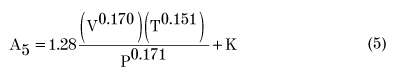where:

A5 = expected number of accidents in five years V = annual average daily traffic T = average daily train traffic P = protection coefficient K = additional parameter

Several states, such as Florida, have developed their own formulae.

A5 can be determined from a set of curves as shown below: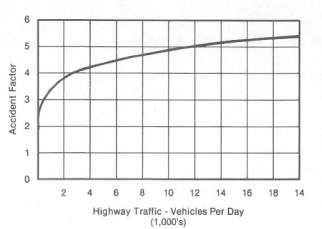Source: Railroad-Highway Grade Crossing Handbook, Second Edition. Washington, DC: U.S. Department of Transportation, Federal Highway Administration, 1986.

Figure 13a. Relation Between Highway Traffic and Accident Factor, VaFigure 13b. Relation Between Railroad Traffic and Accident Factor, T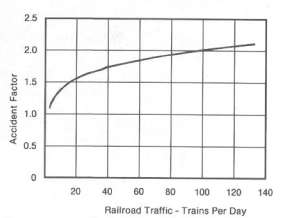Figure 13c. Relation Between Warning Device and Accident Factor, PcThe basic form of the equation for use with these curves is:EXAMPLE: Assume a crossing has an AADT of 3,442 vehicles, an average train traffic of 22 trains per day, and is equipped with wigwags. From Figure 13a, the factor due to highway traffic of 3,442 vehicles per day is found to be 3,99. From Figure 13b, the factor due to train traffic of 22 trains per day is found to be 1.59, and from Figure 13c, the factor for wigwags is found to be 1,99. Substituting these factors into the equation, it is found that the hazard index is equal to:From Figure 13d, K is determined to be + 2.58 for a value of lu of 4.08 and, with this value for the parameter, the expected number of accidents in 5 years is 6.66.

Florida Department of Transportation Accident Prediction Model

The Florida State University developed an accident prediction model for the Florida Department of Transportation. The model was developed using stepwise regression analysis, transformation of data, dummy variables, and transformation of the accident prediction model to its original scale. The resulting model is:where:

A = vehicles per day or annual average daily

traffic L = number of lanes

ln = logarithm to the base e

MASD = actual minimum stopping sight distance along highway

MCSD = clear sight distance (ability to see approaching train along the highway, recorded for the four quadrants established by the intersection of the railroad tracks and road) RSSD = required stopping sight distance on wet pavement

St = maximum speed of train

T = yearly average of the number of trains per day

ta = ln of predicted number of accidents in four-year period at crossings with active traffic control devices

tp= ln of predicted number of accidents in four-year period at crossing with passive traffic control devices

VV = posted vehicle speed limit unless geometrics dictate a lower speed

y = predicted number of accidents per year at crossing

* This variable is omitted if crossing is flagged or the circulation is less than zero.

** This variable is omitted if sight restriction is due to parallel road. *** This variable is omitted when gates are present.

The predicted number of accidents per year, y, is adjusted for accident history as follows:where:

Y = accident prediction adjusted for accident history

y = accident prediction based on the regression model

H = number of accidents for six-year history or since year of last improvement

P = number of years of the accident history period

A simple method of rating each crossing from zero to 90 was derived based mathematically on the accident prediction. This method, entitled Safety (Hazard) Index, is used to rank each crossing. A Safety Index of 70 is considered safe (no further improvement necessary). A Safety Index of 60, or one accident every nine years, would be considered marginal. The Safety Index is calculated as follows:where:

R = safety index

Y = adjusted accident prediction value

X = 90 when less than 10 school buses per day traverse the crossing = 85 when 10 or more school buses per day and active traffic control devices exist without gates = 80 when 10 or more school buses per day and passive traffic control devices exist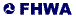United States Department of Transportation - Federal Highway Administration